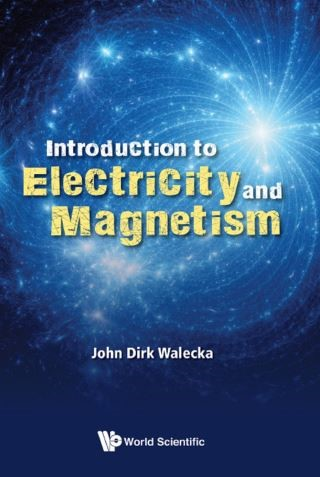## Introduction to Electricity and Magnetism• Description
• Author
• Info
• Reviews

### Description

These lectures provide an introduction to a subject that together with classical mechanics, quantum mechanics, and modern physics lies at the heart of today's physics curriculum. This introduction to electricity and magnetism, aimed at the very best students, assumes only a good course in calculus, and familiarity with vectors and Newton's laws; it is otherwise self-contained. Furthermore, these lectures, although relatively concise, take one from Coulomb's law to Maxwell's equations and special relativity in a lucid and logical fashion.

An extensive set of accessible problems enhances and extends the coverage. Review chapters spaced throughout the text summarize the material. Clear departure points for further study are indicated along the way.

The principles of electromagnetism, as synthesized in Maxwell's equations and the Lorentz force, have such an astonishing range of applicability. A good introduction to this subject, even at the cost of some repetition, allows one to approach the many more advanced texts and monographs with better understanding and a deeper sense of appreciation that both students and teachers can share alike.

Sample Chapter(s)
1. Introduction

Contents:
• Preface
• Electricity:
• Introduction
• Coulomb's Law
• The Electric Field
• Gauss's Law
• The Electrostatic Potential
• Electric Energy
• Capacitors and Dielectrics
• Currents and Ohm's Law
• DC Circuits
• Review of Electricity
• Magnetism:
• Vectors
• The Magnetic Force and Field
• Ampere's Law
• Electromagnetic Induction
• Magnetic Materials
• Time-Dependent Circuits
• Review of Magnetism
• Electromagnetism:
• Maxwell's Equations
• Waves
• Electromagnetic Waves
• More Electromagnetic Waves
• The Theory of Special Relativity
• Review of Electromagnetism
• Problems
• Appendices:
• Significant Names in Electricity and Magnetism
• Fundamental Constants
• Bibliography
• Index

• Provides a clear, self-contained, calculus-based introduction to a subject that together with classical mechanics, quantum mechanics, and modern physics lies at the heart of today's physics curriculum
• Assumes only a good course in calculus and familiarity with vectors and Newton's laws
• Presentation then self-contained
• Takes the reader from Coulomb's law to Maxwell's equations and special relativity in a lucid and logical fashion
• An extensive set of accessible problems enhances and extends the coverage
• Allows one to approach the many more advanced texts and monographs with better understanding and a deeper sense of appreciation